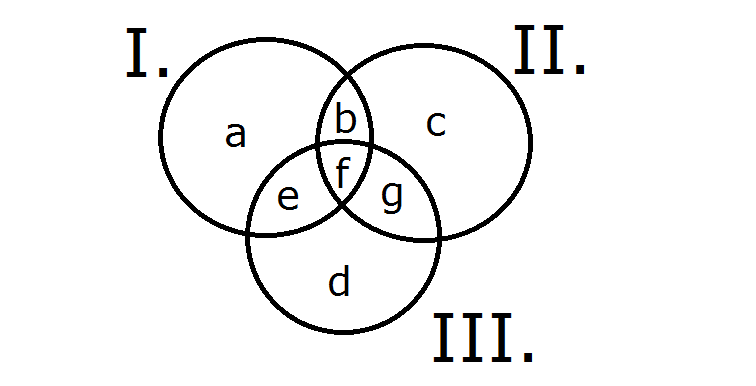Question 11

# In a survey of political preference, 78% of those asked were in favor of at least one of the proposals: I, II and III. 50% of those asked favored proposal I, 30% favored proposal II, and 20% favored proposal III. If 5% of those asked favored all three of the proposals, what percentage of those asked favored more than one of the 3 proposals.

Solution

Let the distribution of votes for each of the proposal be as given below.
From the information given, we know that

a+b+c+d+e+f+g = 78 --- (1)
a+b+e+f = 50  ---- (2)
b+c+f+g = 30 ----  (3)
e+f+g+d = 20 ---- (4) and
f = 5 --- (5)

We need to find b+e+g+f = ?
In the above equations, (2)+(3)+(4) - (1) implies

(a+b+e+f)+(b+c+f+g)+(e+f+g+d) - (a+b+c+d+e+f+g) = 50+30+20-78 = 22
Or, b+e+g+2f=22.
As, f = 5, it implies that b+e+g+f=17